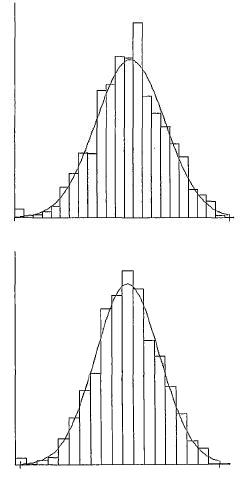# Histogram group number

Sturges formula and other methods of determining the number of groups in histogram generationToday I will talk about statistics and histograms. Generally speaking, the histogram is a graphic display of the grouping method, i.e., distributing the measurement set of some quantity into groups according to an important feature. The grouping method is widely used for primary data processing.

Under the primary data in the statistics, we understand the statistical series, which is called time series when it comes to changing phenomena in time, or variation series when it comes to changing phenomena in time comes to the composition or structure of the investigated phenomenon.

If it comes to the series, based on the qualitative attribute (for example, enterprises by their property category), these series are called attributive, if the series is built by quantitative attribute (for example, enterprises by their trade turnover), they are called variational.

Depending on the continuity of the variation, there are discrete and interval variation series.

A histogram is a bar graph constructed based on the findings, which are divided into several groups. The column's height corresponding expresses the amount of data allocated in each group (frequency) to this group.

The histogram can be built for any series. If it is an attribute or a discrete variation series (for example, the number of workers in each wage category), the number of allocated groups is equal to the number of the characteristic value options. In the interval variation series, the number of groups will depend on the size of the interval used to group data.

Interval - is the difference between the maximum and minimum values of an attribute in each group. i.e., the more groups, the lower the interval and vice versa. Groups, in this case, are sometimes referred to as interval classes.

For example, you can divide the obtained data about the number of workers on the enterprises by the following groups:
up to 25 men
25-50 men
50-100 men
more than 100 men.

Thus, the histogram will contain 5 columns, the height of which will correspond to the number of companies in this group.
We'll note that the distribution above is an example of the uneven intervals use, allocated with the research program, i.e., us.

The question of choosing the value of the interval (number of groups) used for elements of interval variation series grouping is not an easeful one. Apart from the fact that the histogram is an excellent means of data visualization, it is also nothing more than an approximation of probability distribution function (see the picture). I.e., the value of a column in each group shows the probability that the next measured value will fall into this group.

A large number of groups can give too "sharp" graph and the little number - too "smooth". Ideally, it's better to have the number of groups giving you the least deviations of the probability distribution function, i.e., giving you the most precise evaluations of the studied phenomenon's true probability distribution function.

The mathematicians studied this.

It seems first one was Sturges. He reviewed the idealized frequency histogram of k classes, where the i-th value equal to the binomial coefficient . For sufficiently large k the histogram form was approaching the form of a normal distribution. The sum of all values was equal .

Thus, for n results of the value measurement that are normally distributed, the number of classes used in the histogram should be taken as and the form of the obtained histogram will be closer to the shape of the normal distribution for sufficiently large k. This is the Sturgess formula. In this form, it has got almost all the textbooks on statistics.
This formula is now being criticized because it explicitly uses the binomial distribution to approximate the normal distribution, which is not always applicable. It is believed that this formula allows you to build a satisfactory histogram if the number of dimensions less than 200.

There are several alternative formulas, some of which calculate the length of the interval and then determine the number of required classes (seehere).

Let's review some of these formulas.

Scott's formula
, where h - interval length, s - the standard deviation of the measurement series

Friedman/Diaconis formula
, where h - interval length, (IQ) - the difference between the upper and lower quartile.

These formulas are quite simple and justified by statistical theory and considered more preferable to Sturgess.

The calculator below uses as the results the measurements of random number generator built into Javascript.
Since the generator's distribution function is practically constant, the random number received from the generator can be further modified by selecting something interesting in the "Function ..." graph. This will actually let us observe more cheerful graphics instead of almost a straight line.

In addition to constructing a histogram using the number of classes obtained by the Sturgess formula, it builds histograms based on the number of classes based on Scott and Friedman/Diaconis and the number of classes randomly set by the user.

Of course, there is no practical application to this calculator, but you can see the difference in the number of classes and the histogram's appearance.#### Sturgess formula and other methods of determining the number of groups in histogram generation

Number of randomly generated observation results
Function modifying the results of random number generator
Digits after the decimal point: 2
Number of classes according to Sturgess

Grouping using the Sturgess formula
The file is very large. Browser slowdown may occur during loading and creation.
Average

Standard deviation

Number of classes according to Scott

Scott
The file is very large. Browser slowdown may occur during loading and creation.
First quartile

Third quartile

Number of classes according to Friedman/Diaconis

Friedman/Diaconis
The file is very large. Browser slowdown may occur during loading and creation.